###### INFORMATION

Psicothema was founded in Asturias (northern Spain) in 1989, and is published jointly by the Psychology Faculty of the University of Oviedo and the Psychological Association of the Principality of Asturias (Colegio Oficial de Psicólogos del Principado de Asturias).
We currently publish four issues per year, which accounts for some 100 articles annually. We admit work from both the basic and applied research fields, and from all areas of Psychology, all manuscripts being anonymously reviewed prior to publication.

###### PSICOTHEMA
• Director: Laura E. Gómez Sánchez
• Frequency:
February | May | August | November
• ISSN: 0214-9915
• Digital Edition:: 1886-144X
• Address: Ildelfonso Sánchez del Río, 4, 1º B
33001 Oviedo (Spain)
• Phone: 985 285 778
• Fax: 985 281 374
• Email:psicothema@cop.es

Psicothema, 2000. Vol. Vol. 12 (Suplem.2). 382-385

### SUGGESTIONS FOR COPING WITH MULTICOLLINEARITY IN CONDENSATION LOGISTIC RULES

Josep L. Melià

University of Valencia

Whitely (1980) proposed the first conjunctive linkage rule relating subtask probabilities to the total item response. De Boeck (1991) defines two main families of linking rules: (1) the compensatory rule, or weighted additive and (2) the conjunctive rule. The latter formally includes the (3) disjunctive rule that can also be formulated as a conjunctive rule of the errors. Afterwards, De Boeck (1993) suggested two mixed logistic linking rules: the partially conjunctive and the partially disjunctive rules. In this paper I present a combined logistic linking rule that summarizes the three classes of effects: conjunctive, disjunctive and compensatory. The analysis of multicollinearity among the three terms, in the two components case, allows the reduction of the partially conjunctive, the partially disjunctive and the conjunctive-disjunctive-compensatory rules into a more simple combined logistic rule.

Análisis de la multicolinealidad. Whitely (1980) propuso la primera regla de enlace conjuntiva enlazando las probabilidades de las subtareas con la respuesta total del ítem. De Boeck (1991) ha definido dos familias principales de reglas de enlace (1) la regla compensatoria, o aditiva ponderada, y (2) la regla conjuntiva. Esta última incluye formalmente a la (3) regla disyuntiva, que puede formularse como una regla conjuntiva de los errores. Posteriormente De Boeck (1993) sugirió dos reglas logísticas de enlace de carácter mixto: la parcialmente conjuntiva y la parcialmente disyuntiva. En este trabajo presento una regla logística combinada que resume las tres clases de efectos, conjuntivos, disyuntivos y compensatorios. El análisis de la multicolinealidad entre los tres términos, para el caso de dos componentes, permite la reducción de las reglas parcialmente conjuntiva, parcialmente disyuntiva y conjuntiva-disyuntiva-compensatoria en una regla logística combinada más simple.

The development of psychometrics as a science engaged with the design of models of psychological processing (Samejima 1997) has made componential IRT models one of the more promising branches of its improvement.

Instead of postulating a single latent trait, componential latent trait models attempt to identify the information processing components that are involved in test performance.

Componential models can differ on three major respects: (1) the IRT function applied to the components, (2) the dimensionality, and (3) the relationship between the components.

In the Multicomponent Latent Trait Models (MLTM) the probability of giving a certain answer to the total item is expressed as a function of the probability of giving certain responses to the component processing events (Whitely, 1980; Embretson, 1997).

The IRT function links the probability of success in each component to the person and the item parameters. Generally, each component follows the Rasch model, as shown in Equation 1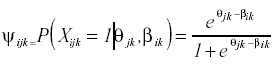(1)

An outstanding example of unidimensional componential model is the Linear Logistic Trait Model (LLTM; Fischer, 1973). In this case, the probability of success is expressed as a function of a linear combination of item difficulty factors and a single person ability parameter. If each component implies a latent trait, as in the MLTM, the model is multidimensional. Whitely (1980) uses a Rasch model to estimate the probability of success in each component, then combines these probabilities using a conjunctive rule and a guessing parameter, and contrasts the model, comparing observed response frequencies (or probabilities) on the total item with the response frequencies (or probabilities) predicted by the multicomponent model.

A condensation rule shows the relationship between the observed random variables and a set of latent random variables (Maris, 1995). In componential models, a condensation rule may connect (1) components, (2) properties of the items, or (3) dimensions, to the total item answers.

Once the total item is conceived as a set of subtasks that define components, a componential rule linking the subtasks to the composite task is needed. If the componential model linkage rule combines components, it can work over two classes of component elements: (1) subtask probabilities, usually obtained by a Rasch Model on the subtask, or (2) subtask indicators, such as subtask scores applied to subtasks responses.

Whitely (1980) proposed the first conjunctive linkage rule (Equation 2)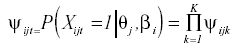(2)

in which the probability of success of the person j on the total item i is modeled as the product of the probabilities of success in each of the k subtasks as defined by equation 1.

The De Boeck contribution

De Boeck (1991) defines three families of linking rules: (1) the compensatory rule, or weighted additive and (2) the conjunctive rule. The latter formally includes the (3) disjunctive rule.

The compensatory rule implies that the parameters of the subtasks have an additive contribution to the probability of success in the composite task. This means, in the words of De Boeck (1991) that «the subtasks have only main effects» and implies that the contributions from the different subtasks can compensate each other.

The conjunctive rule supposes that success in the composite task implies success in each and every one of the subtasks.

The disjunctive rule supposes that success in any subtask is a sufficient condition for success in the composite task. But, as De Boeck (1991) observes, this can also be formulated as a conjunctive rule of the errors.

Conjunctive and disjunctive rules assume a deterministic relationship between the composite task and it’s subtasks.

The use of logistic functions to connect partial results to the total probabilities is well known (Aldrich and Nelson, 1984). De Boeck (1993) suggests the partially conjunctive model and the partially disjunctive model as a way to unite the compensatory and conjunctive, or disjunctive, effects into a logistic linking rule.

The partially conjunctive model as formulated by De Boeck (1993) is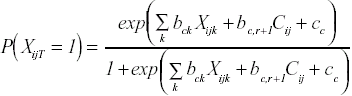(3)

where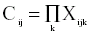expresses the conjunctive nature of the model.

The partially disjunctive model is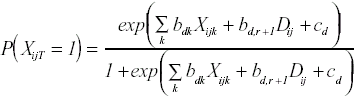(4)

where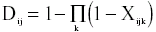is the disjunctive term.

From the point of view of the analysis of componential data, De Boeck (1993) suggests to adjust the conjunctive-compensatory function and, depending on the magnitude and sign of the weights, to reduce this model or introduce the disjunctive term.

The conjunctive-disjunctive-compensatory condensation rule

Following De Boeck’s (1993) work, as the c constants can be integrated into one term, it can be observed that if bc,r+1 = bd,r+1 = 0, both models, the partially conjunctive model and the partially disjunctive model, become equal, both reduced to the compensatory model.

This suggests the formulation of a conjunctive-disjunctive-compensatory model that summarizes the three classes of effects. Simplifying the notation, the model can be written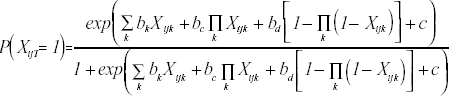(5)

This model is a theoretical formulation that can be used to express, together, the three classes of effects.

But this formulation allows us to discuss the relationships between the terms compensatory, conjunctive and disjunctive and obtains a simplified expression for the logistic rule.

If, as usual, the composite task is modeled by a Rasch Model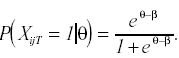It follows that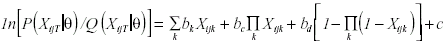or, in an equivalent expressionThe compensatory, conjunctive and disjunctive effects can be understood as linear in the logit of the probability of success in the total task.

It suggests (1) the convenience of a formulation of the model in logits for analyzing the effects of the components [as has been done by Janssen and De Boeck (1997)], and (2) the analogy between the MLTM model and the LLTM model under three conditions: (a) the transformation of the MLTM linking rule into a logistic condensation rule, (b) a binary sound structure of the composite items, and (c) a Rasch model governing the composite item. The third condition implies the reduction of the model into a unidimensional one.

Avoiding multicollinearity

The formulation of the conjunctive-disjunctive-compensatory linking rule in the linear form reveals the presence of multicollinearity between the conjunctive, disjunctive and compensatory terms. The analysis of this multicollinearity will provide the simplification of the linking rule.

Suppose an item or composed task, XT, that exclusively and exhaustively depends on two subtasks XA and XB. For simplicity, we call the composed item T and the components A and B. Assume that T, is dichotomous and governed by a Rasch Model. It is unnecessary to suppose that A and B are dichotomous or that a Rasch Model can be used on them. The two components case is useful for this analysis because its conclusions can be extrapolated, without loss of generality, to other multicomponent models and because this is the more frequent case in the application of multicomponent models.

Using this nomenclature for the two component case the basic equation may be written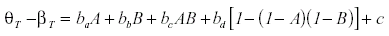The multicollinearity between the terms A and B and their product AB is very high. To eliminate this multicollinearity, the same strategy that is used in moderated multiple regression can be used. The purpose of this strategy is to obtain a corrected interaction term, AB*, that is linearly independent from A and B. To do this, we need to estimate the regression of the interaction term, AB, on the component terms A and B: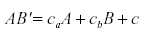The regression weights ca and cb are necessary to provide the corrected interaction term AB*:

AB* = ( A - cb ) ( B - ca )

The term AB* is linearly independent from the component terms A and B

r (AB*,A) = R(AB*,B) = 0

and expresses the conjunctive interaction effects without multicollinearity. The corrected conjunctive interaction term, AB*, can be incorporated into the equation along with the compensatory effects from A and B.

In the same way, the multicollinearity between the disjunctive term, [ 1 - ( 1 - A ) ( 1 - B ) ], and the component terms A and B requires a disjunctive interaction term that is linearly independent from A and B. To obtain this term, the disjunctive term is regressed on the component terms:

[ 1 - ( 1 - A ) ( 1 - B ) ] ’ = ca’ A + c b’ B + c’.

The corrected disjunctive term AB** is

AB** = [ 1 - ( 1 - A - Cb’ ) ( 1 - B - ca’ ) ].

The new corrected term, AB**, expresses the disjunctive interaction effects free from any linear relationship with A and B,

r(AB**,A) = R(AB**,B) = 0

For this reason the term AB** can be added to the compensatory effects in the equation.

Nevertheless, once we have the conjunctive corrected effects, AB*, and the disjunctive corrected effects, AB**, it can be seen that these terms are linearly redundant. In fact, the relationship is:

AB** = 1 - AB*.

A simplified combined logistic rule

The disjunctive term AB** is unnecessary and the linking rule may be reduced, without loss of information, to the following form:

θT - βT = baA + bbB + bcAB* + c

where ba is the weight of the component A, bb is the weight of the component B, and bc is the weight of the conjunctive-disjunctive effects AB*.

The condensation rule may be written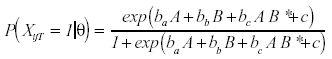This condensation logistic rule is a regression form that resembles the LLTM. However, the LLTM expresses the probability of success as a function of the person parameter and a linear combination of item difficulty factors. The condensation logistic rule works directly over the component scores, but introduces an interaction term that represents the conjunctive-disjunctive effects.

An expression such as

Y' = baA + bbB + bc ( A - cb )( B - ca ) + c

is characteristic of the continuous moderated regression (e.g., Aguinis and Stone-Romero, 1997; DeShon and Alexander, 1996; Zedeck, 1971) and has been explored in several areas of psychological measurement with the purpose of finding moderator variables that increase predictive validity.

In general, research using moderated regression has produced very few successful contributions, but there is still little evidence with respect to interaction effects on the logistic componential rules.

Aguinis, H. & Stone-Romero, E.F. (1997). Methodological artifacts in moderated multiple regression and their effects on statistical power. Journal of Applied Psychology, 82(1), 192-206.

Aldrich, J. H. & Nelson, F. D. (1984). Linear probability, logit and probit models. Beverly Hills: Sage.

De Boeck, P. (1991). Componential IRT models. Unpublished manuscript. University of Leuven. Belgium.

De Boeck, P. (1993). Componential linkage models based on subtask response. In Steyer, R,, Wender, K. F., & Widaman, K. F. (Eds.), Psychometric methodology. Proceedings of the 7th European meeting of the Psychometric Society (pp. 110-113). New York: Gustav Fischer Verlag.

DeShon, R. P. & Alexander, R. A. (1996). Alternative procedures for testing regression slope homogeneity when group error variances are unequal. Psychological Methods, 1(3), 261-277.

Embretson, S. E. (1997). Multicomponent response models. In van der Linden, W. J. & Hambleton, R.K. (Eds.) Handbook of modern item response theory (pp. 305-321). New York: Springer- Verlag.

Fischer, G. H. (1973). The linear logistic test model as an instrument in educational research. Acta Psychologica, 37, 359-374.

Janssen, R. & De Boeck, P. (1997). Psychometric modeling of componentially designed synonym tasks. Applied Psychological Measurement, 21(1), 37-50.

Maris, E. (1995). Psychometric latent response models. Psychometrika, 60(4), 523-547.

Samejima, F. (1997). Departure from normal assumptions: A promise for future psychometrics with substantive mathematical modeling. Psychometrika. 62(4), 471-493.

Whitely, S. E. (1980.). Multicomponent latent-trait models for ability test. Psychometrika., 45(4), 479-494.

Zedeck, S. (1971). Problems with the use of «moderator«» variables. Psychological Bulletin, 76(4), 295-310.